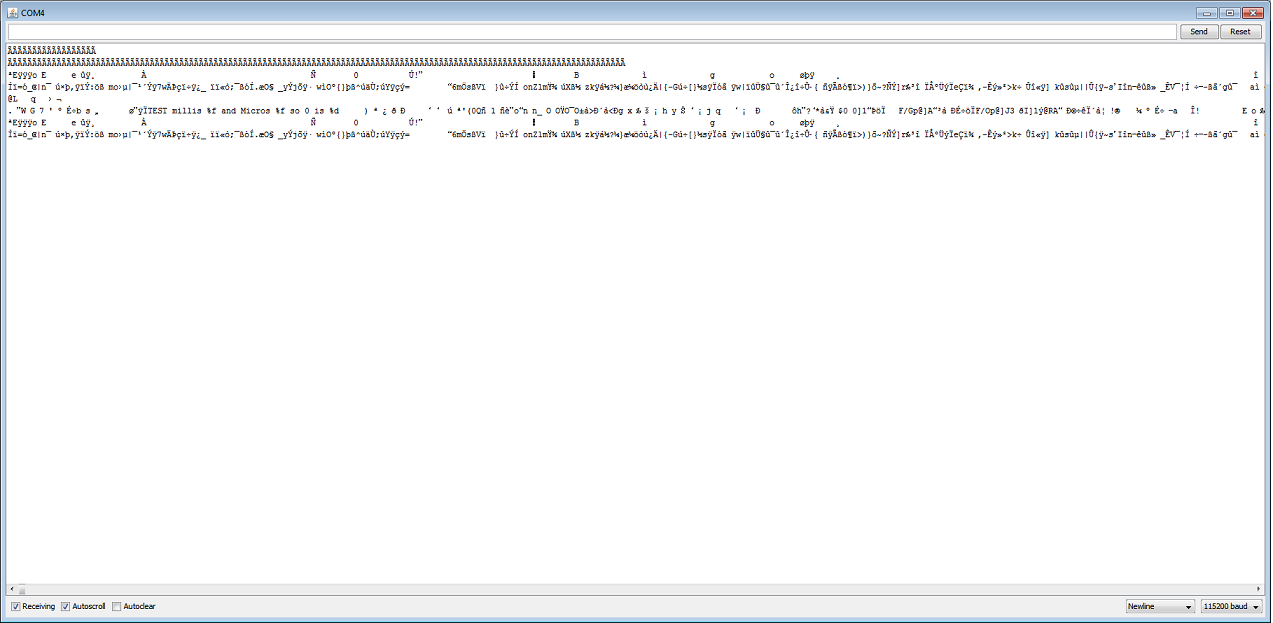sprintf on Mega 2560 not working

Hello, when i use sprintf and Serial stuff at once, everything getting screwed When i run this code:

void setup()
{
Serial.begin(115200);
}

char* s;

void loop()
{

sprintf(s,"TEST millis %f and Micros %f so 0 is %d",millis(),micros(),0);
Serial.println(s);
s = "";

delay(1000);
}

This output happens (Spam, very fast.. the 1000milliseconds delay will be ignored):Serial stuff is working without sprintf

Informations: im using Arduini 1.0.4 IDE i tested this code on Arduino UNO R2 and on my both MEGA 2560

A year ago, it worked well (i think it was arduino 1.0 IDE), since 1.0.1 its screwed

And before you think the %f in sprintf causing this problem, i tried to floor/round the millis() and micros() command to use it with %d and/or %i .. no luck

Does anyone have a fix for this? maybe a working stdio.h?

void setup()
{
Serial.begin(115200);
}

char s;  // <<<<<<<<<<<<<<<<<<<<<<<<<<<<<<<<<<<<<<<<

void loop()
{

sprintf(s,"TEST millis %ul and Micros %ul so 0 is %d",millis(),micros(),0);  // <<<<<<<<<<<<<<<<<<<<<<<<<<<<<<<<<<<<<<<<
Serial.println(s);

delay(1000);
}

You failed to provide an actual buffer. %f has never worked. %f never will work when you pass an unsigned long.